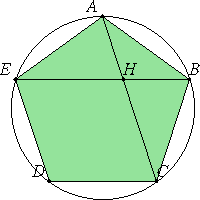# Proposition 8

If in an equilateral and equiangular pentagon straight lines subtend two angles are taken in order, then they cut one another in extreme and mean ratio, and their greater segments equal the side of the pentagon.

In the equilateral and equiangular pentagon ABCDE let the straight lines AC and BE, cutting one another at the point H, subtend two angles taken in order, the angles at A and B.

I say that each of them has been cut in extreme and mean ratio at the point H, and their greater segments equal the side of the pentagon.IV.14

Circumscribe the circle ABCDE about the pentagon ABCDE.

I.4

Then, since the two straight lines EA and AB equal the two lines AB and BC, and they contain equal angles, therefore the base BE equals the base AC, the triangle ABE equals the triangle ABC, and the remaining angles equal the remaining angles respectively, namely those opposite the equal sides.

I.32

Therefore the angle BAC equals the angle ABE. Therefore the angle AHE is double the angle BAH.

But the angle EAC is also double the angle BAC, for the circumference EDC is also double the circumference CB.

I.6

Therefore the angle HAE equals the angle AHE. Hence the straight line HE also equals EA, that is, AB.

I.5

And, since the straight line BA equals AE, therefore the angle ABE also equals the angle AEB.

But the angle ABE was proved equal to the angle BAH, therefore the angle BEA also equals the angle BAH.

I.32

And the angle ABE is common to the two triangles ABE and ABH, therefore the remaining angle BAE equals the remaining angle AHB. Therefore the triangle ABE is equiangular with the triangle ABH.

VI.4

Therefore, proportionally EB is to BA as AB is to BH.

But BA equals EH, therefore BE is to EH as EH is to HB.

VI.14

And BE is greater than EH, therefore EH is also greater than HB.

Therefore BE has been cut in extreme and mean ratio at H, and the greater segment HE equals the side of the pentagon.

Similarly we can prove that AC has also been cut in extreme and mean ratio at H, and its greater segment CH equals the side of the pentagon.

Therefore, if in an equilateral and equiangular pentagon straight lines subtend two angles are taken in order, then they cut one another in extreme and mean ratio, and their greater segments equal the side of the pentagon.

Q.E.D.

## Guide

This proposition shows why cutting a line in extreme and mean ratio is so important.

#### Use of this proposition

This proposition is used in the proof of XIII.11 to establish that the side of a regular pentagon inscribed in a circle with rational diameter is the irrational straight line called minor.